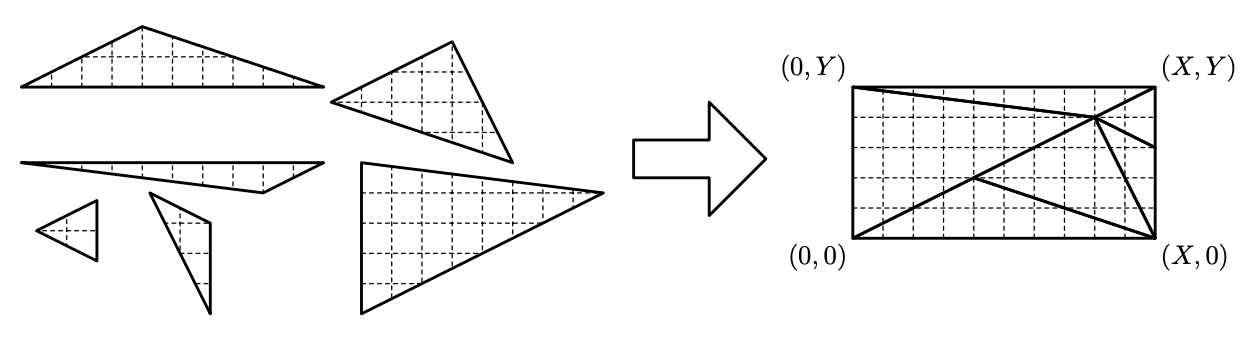시간 제한 메모리 제한 제출 정답 맞은 사람 정답 비율
2 초 512 MB 0 0 0 0.000%

## 문제

During the recent two-day storm, a group of sailors shipwrecked on a desert island in the Mediterranean sea. Since their radio is broken, they cannot call for help and their only chance of survival is escaping on a raft. However, they need a sail to power the raft. Luckily, they recovered the main sail from the wrecked ship, but it is torn to several shreds. Hence, they have to sew the shreds together to obtain the original sail.

The sailors quickly realized that each of the shreds is a triangle. Because there were stripes on the sail, it was easy to identify the correct orientation of its triangular parts. In fact, they have to respect the orientations of the shreds, since otherwise the sail would be less resistant to wind and might be torn again. They were trying to find the original arrangement for several hours but with no result. They think that a computer can help them and asked you for help.

You are given the description of the triangular shreds (given by the plane coordinates of the vertices of each triangle) and the dimensions of the original sail which was a rectangle (given by two integers X and Y ). Your task is to move the shreds (without rotation) to reconstruct the original sail (see the figure). In particular, the triangles should be moved in such a way that

• the whole rectangular area is covered,
• no two triangles intersect, i.e., there is no point which is contained in the interiors of two different triangles, and
• there is no triangle whose part lies outside of the rectangle.

You may assume that there exists a solution for each of the input data. In particular, it holds that the sum of the areas of the shreds is equal to the area of the original sail. There can be several shreds of the same shape.## 입력

Each of the input files describe a single test case and has the following structure. The first line contains three integers N, X, and Y, separated by single spaces. X and Y (1 ≤ X, Y ≤ 1 000 000) determine the dimensions of the original sail, X being the width and Y being the height, and N, 2 ≤ N ≤ 1 000, is the number of triangular shreds of the sail.

The next N lines describe the individual triangles: (i + 1)-th line contains six integers Ai,x, Ai,y, Bi,x, Bi,y, Ci,x and Ci,y separated by single spaces. These six numbers describe the three vertices Ai, Bi, and Ci of the i-th triangle: the vertex Ai has coordinates (Ai,x, Ai,y), Bi has coordinates (Bi,x, Bi,y), and Ci has coordinates (Ci,x, Ci,y). You may assume that 0 ≤ Ai,x, Ai,y, Bi,x, Bi,y, Ci,x, Ci,y ≤ 1 000 000.

Note that the position of a triangle (specified in the input file) is irrelevant. E.g., if the third line of the example input were replaced with 6 6 14 5 16 6, the given set of the triangular shreds would be the same.

## 출력

The output should consist of exactly N lines describing the arrangement of the shreds of the sail such that all the three conditions in the task description are met. More specifically, the i-th line should contain two integers, A*i,x and A*i,y separated by a single space—the coordinates of the point Ai after moving the i-th shred to the appropriate place. Note that the left bottom corner of the sail has always coordinates (0, 0) and the right top has coordinates (X, Y) as depicted in the figure.

## 채점 및 기타 정보

• 예제는 채점하지 않는다.